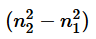Courses

# Practice Exercise - 1(Structure Of Atom - JEE Mains)

## 20 Questions MCQ Test | Practice Exercise - 1(Structure Of Atom - JEE Mains)

Description
This mock test of Practice Exercise - 1(Structure Of Atom - JEE Mains) for helps you for every entrance exam. This contains 20 Multiple Choice Questions for Practice Exercise - 1(Structure Of Atom - JEE Mains) (mcq) to study with solutions a complete question bank. The solved questions answers in this Practice Exercise - 1(Structure Of Atom - JEE Mains) quiz give you a good mix of easy questions and tough questions. students definitely take this Practice Exercise - 1(Structure Of Atom - JEE Mains) exercise for a better result in the exam. You can find other Practice Exercise - 1(Structure Of Atom - JEE Mains) extra questions, long questions & short questions for on EduRev as well by searching above.
QUESTION: 1

Solution:
QUESTION: 2

Solution:
QUESTION: 3

### If in the hydrogen atom P.E. at ∞ is choosen to be 13.6 eV then the ratio of T.E. to K.E. for 1st orbit of H-atom is __________ .

Solution:
QUESTION: 4

The light radiations with discrete quantities of energy are called ___________.

Solution:
QUESTION: 5

The ratio of the energy of a photon of 2000 Å wavelength radiation to that of 4000 Å radiation is

Solution:
QUESTION: 6

The energy of electron is maximum at :

Solution:
QUESTION: 7

Which electronic level would allow the hydrogen atom to absorb a photon but not to emit a photon:

Solution:
QUESTION: 8

The third line in Balmer series corresponds to an electronic transition between which Bohr’s orbits in hydrogen :

Solution:
QUESTION: 9

The orbital angular momentum of an electron in 2s orbital is :

Solution:
QUESTION: 10

Which quantum number is not related with Schrodinger equation :

Solution:
QUESTION: 11

The shortest wavelength of He atom in Balmer series is x, then longest wavelength in the Paschene series of Li+2 is :

Solution:
QUESTION: 12

An electron in a hydrogen atom in its ground state absrobs energy equal to the ionisation energy of Li+2. The wavelength of the emitted electron is

Solution:
QUESTION: 13

An electron, a proton and an alpha particle have kinetic energies of 16E, 4E and E respectively. What is the qualitative order of their de-Broglie wavelengths?

Solution:
QUESTION: 14

Given ΔH for the process Li(g) → Li+3 (g) + 3e- is 19800 kJ/mole & IE1 for Li is 520 then IE2 & IE3 of Li+ are respectively (approx, value) :

Solution:
QUESTION: 15

The ratio of difference in wavelengths of 1st and 2nd lines of Lyman series in H–like atom to difference in wavelength for 2nd and 3rd lines of same series is :

Solution:
QUESTION: 16

If radius of second stationary orbit (in Bohr’s atom) is R. Then radius of third orbit will be

Solution:
QUESTION: 17

The ratio of wave length of photon corresponding to the α - line of lyman series in H-atom and β - line of Baimer series in He+ is

Solution:
QUESTION: 18

Three energy levels P, Q, R of a certain atom are such that EP < EQ < ER. If λ1, λ2, and λ3, are the wave length of radiation corresponding to transition R → Q : Q → P and R → P respectively. The correct relationship between λ1, λ2 and λ3 is

Solution:
QUESTION: 19

The Value of (n2 + n1) andfor He+ ion in atomic spectrum are 4 and 8 respectively. The wavelength of emitted photon when electron jump from n2 to n1 is

Solution:
QUESTION: 20

Number of possible spectral lines which may be emitted in bracket series in H atom if electrons present 9th  excited level returns to ground level, are

Solution: